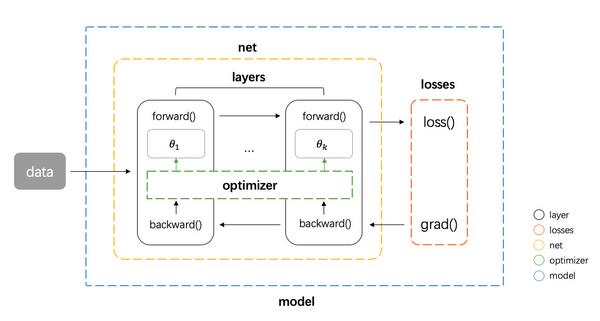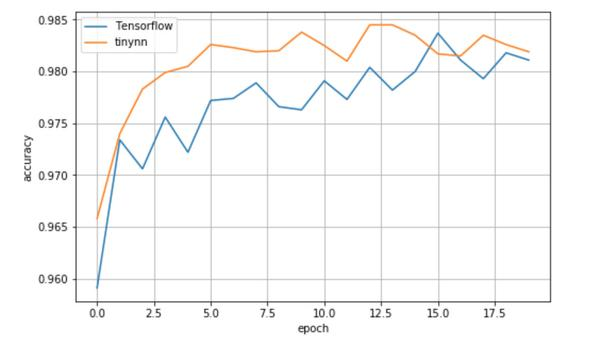• 问答
• 技术
• 实践
• 资源

1. 组件抽象
2. 组件实现
3. 整体结构
4. MNIST 例子
5. 总结
6. 附录
7. 参考

组件抽象

1. 数据在网络层之间的流动：前向传播和反向传播可以看做是张量 Tensor（多维数组）在网络层之间的流动（前向传播流动的是输入输出，反向传播流动的是梯度），每个网络层会进行一定的运算，然后将结果输入给下一层
2. 计算损失：衔接前向和反向传播的中间过程，定义了模型的输出与真实值之间的差异，用来后续提供反向传播所需的信息
3. 参数更新：使用计算得到的梯度对网络参数进行更新的一类计算

• tensor 张量，这个是神经网络中数据的基本单位
• layer 网络层，负责接收上一层的输入，进行该层的运算，将结果输出给下一层，由于 tensor 的流动有前向和反向两个方向，因此对于每种类型网络层我们都需要同时实现 forward 和 backward 两种运算
• loss 损失，在给定模型预测值与真实值之后，该组件输出损失值以及关于最后一层的梯度（用于梯度回传）
• optimizer 优化器，负责使用梯度更新模型的参数

• net 组件负责管理 tensor 在 layers 之间的前向和反向传播，同时能提供获取参数、设置参数、获取梯度的接口
• model 组件负责整合所有组件，形成整个 pipeline。即 net 组件进行前向传播 -> losses 组件计算损失和梯度 -> net 组件将梯度反向传播 -> optimizer 组件将梯度更新到参数。组件实现

# define model
net = Net([layer1, layer2, ...])
model = Model(net, loss_fn, optimizer)

# training
pred = model.forward(train_X)

# inference
test_pred = model.forward(test_X)

tensor

tensor 张量是神经网络中基本的数据单位，我们这里直接使用 numpy.ndarray 类作为 tensor 类的实现

layer

# layer.py
class Layer(object):
def __init__(self, name):
self.name = name

def forward(self, inputs):
raise NotImplementedError

raise NotImplementedError

# layer.py
class Dense(Layer):
def __init__(self, num_in, num_out,
w_init=XavierUniformInit(),
b_init=ZerosInit()):
super().__init__("Linear")

self.params = {
"w": w_init([num_in, num_out]),
"b": b_init([1, num_out])}

self.inputs = None

def forward(self, inputs):
self.inputs = inputs
return inputs @ self.params["w"] + self.params["b"]

# layer.py
class Activation(Layer):
"""Base activation layer"""
def __init__(self, name):
super().__init__(name)
self.inputs = None

def forward(self, inputs):
self.inputs = inputs
return self.func(inputs)

def func(self, x):
raise NotImplementedError

def derivative_func(self, x):
raise NotImplementedError

class ReLU(Activation):
"""ReLU activation function"""
def __init__(self):
super().__init__("ReLU")

def func(self, x):
return np.maximum(x, 0.0)

def derivative_func(self, x):
return x > 0.0

net

# net.py
class Net(object):
def __init__(self, layers):
self.layers = layers

def forward(self, inputs):
for layer in self.layers:
inputs = layer.forward(inputs)
return inputs

for layer in reversed(self.layers):

for layer in self.layers:

def get_parameters(self):
return [layer.params for layer in self.layers]

def set_parameters(self, params):
for i, layer in enumerate(self.layers):
for key in layer.params.keys():
layer.params[key] = params[i][key]

losses

# loss.py
class BaseLoss(object):
def loss(self, predicted, actual):
raise NotImplementedError

raise NotImplementedError

class CrossEntropyLoss(BaseLoss):
def loss(self, predicted, actual):
m = predicted.shape
exps = np.exp(predicted - np.max(predicted, axis=1, keepdims=True))
p = exps / np.sum(exps, axis=1, keepdims=True)
nll = -np.log(np.sum(p * actual, axis=1))
return np.sum(nll) / m

m = predicted.shape

optimizer

# optimizer.py
class BaseOptimizer(object):
def __init__(self, lr, weight_decay):
self.lr = lr
self.weight_decay = weight_decay

step = list()
# compute step
p = 0
for param in params:
layer = dict()
for k, v in param.items():
block = np.prod(v.shape)
_step = flatten_step[p:p+block].reshape(v.shape)
_step -= self.weight_decay * v
layer[k] = _step
p += block
step.append(layer)
return step

raise NotImplementedError

def __init__(self, lr=0.001, beta1=0.9, beta2=0.999,
eps=1e-8, weight_decay=0.0):
super().__init__(lr, weight_decay)
self._b1, self._b2 = beta1, beta2
self._eps = eps

self._t = 0
self._m, self._v = 0, 0

self._t += 1
self._m = self._b1 * self._m + (1 - self._b1) * grad
self._v = self._b2 * self._v + (1 - self._b2) * (grad ** 2)
# bias correction
_m = self._m / (1 - self._b1 ** self._t)
_v = self._v / (1 - self._b2 ** self._t)
return -self.lr * _m / (_v ** 0.5 + self._eps)

model

# model.py
class Model(object):
def __init__(self, net, loss, optimizer):
self.net = net
self.loss = loss
self.optimizer = optimizer

def forward(self, inputs):
return self.net.forward(inputs)

def backward(self, preds, targets):
loss = self.loss.loss(preds, targets)
params = self.net.get_parameters()
return loss, step

for k, v in param.items():

整体结构

tinynn
├── core
│   ├── initializer.py
│   ├── layer.py
│   ├── loss.py
│   ├── model.py
│   ├── net.py
│   └── optimizer.py

MNIST 例子

• 数据集：MNIST
• 任务类型：多分类
• 网络结构：三层全连接 INPUT(784) -> FC(400) -> FC(100) -> OUTPUT(10)，这个网络接收 $(N, 784)$ 的输入，其中 $N$ 是每次输入的样本数，784 是每张 $(28, 28)$ 的图像展平后的向量，输出维度为 $(N, 10)$ ，其中 $N$ 是样本数，10 是对应图片在 10 个类别上的概率
• 激活函数：ReLU
• 损失函数：SoftmaxCrossEntropy
• batch_size：128
• Num_epochs：20

# example/mnist/run.py
net = Net([
Dense(784, 400),
ReLU(),
Dense(400, 100),
ReLU(),
Dense(100, 10)
])

iterator = BatchIterator(batch_size=args.batch_size)
evaluator = AccEvaluator()
for epoch in range(num_ep):
for batch in iterator(train_x, train_y):
# training
pred = model.forward(batch.inputs)
# evaluate every epoch
test_pred = model.forward(test_x)
test_pred_idx = np.argmax(test_pred, axis=1)
test_y_idx = np.asarray(test_y)
res = evaluator.evaluate(test_pred_idx, test_y_idx)
print(res)

# tinynn
Epoch 0      {'total_num': 10000, 'hit_num': 9658, 'accuracy': 0.9658}
Epoch 1      {'total_num': 10000, 'hit_num': 9740, 'accuracy': 0.974}
Epoch 2      {'total_num': 10000, 'hit_num': 9783, 'accuracy': 0.9783}
Epoch 3      {'total_num': 10000, 'hit_num': 9799, 'accuracy': 0.9799}
Epoch 4      {'total_num': 10000, 'hit_num': 9805, 'accuracy': 0.9805}
Epoch 5      {'total_num': 10000, 'hit_num': 9826, 'accuracy': 0.9826}
Epoch 6      {'total_num': 10000, 'hit_num': 9823, 'accuracy': 0.9823}
Epoch 7      {'total_num': 10000, 'hit_num': 9819, 'accuracy': 0.9819}
Epoch 8      {'total_num': 10000, 'hit_num': 9820, 'accuracy': 0.982}
Epoch 9      {'total_num': 10000, 'hit_num': 9838, 'accuracy': 0.9838}
Epoch 10     {'total_num': 10000, 'hit_num': 9825, 'accuracy': 0.9825}
Epoch 11     {'total_num': 10000, 'hit_num': 9810, 'accuracy': 0.981}
Epoch 12     {'total_num': 10000, 'hit_num': 9845, 'accuracy': 0.9845}
Epoch 13     {'total_num': 10000, 'hit_num': 9845, 'accuracy': 0.9845}
Epoch 14     {'total_num': 10000, 'hit_num': 9835, 'accuracy': 0.9835}
Epoch 15     {'total_num': 10000, 'hit_num': 9817, 'accuracy': 0.9817}
Epoch 16     {'total_num': 10000, 'hit_num': 9815, 'accuracy': 0.9815}
Epoch 17     {'total_num': 10000, 'hit_num': 9835, 'accuracy': 0.9835}
Epoch 18     {'total_num': 10000, 'hit_num': 9826, 'accuracy': 0.9826}
Epoch 19     {'total_num': 10000, 'hit_num': 9819, 'accuracy': 0.9819}

# Tensorflow 1.13.1
Epoch 0      {'total_num': 10000, 'hit_num': 9591, 'accuracy': 0.9591}
Epoch 1      {'total_num': 10000, 'hit_num': 9734, 'accuracy': 0.9734}
Epoch 2      {'total_num': 10000, 'hit_num': 9706, 'accuracy': 0.9706}
Epoch 3      {'total_num': 10000, 'hit_num': 9756, 'accuracy': 0.9756}
Epoch 4      {'total_num': 10000, 'hit_num': 9722, 'accuracy': 0.9722}
Epoch 5      {'total_num': 10000, 'hit_num': 9772, 'accuracy': 0.9772}
Epoch 6      {'total_num': 10000, 'hit_num': 9774, 'accuracy': 0.9774}
Epoch 7      {'total_num': 10000, 'hit_num': 9789, 'accuracy': 0.9789}
Epoch 8      {'total_num': 10000, 'hit_num': 9766, 'accuracy': 0.9766}
Epoch 9      {'total_num': 10000, 'hit_num': 9763, 'accuracy': 0.9763}
Epoch 10     {'total_num': 10000, 'hit_num': 9791, 'accuracy': 0.9791}
Epoch 11     {'total_num': 10000, 'hit_num': 9773, 'accuracy': 0.9773}
Epoch 12     {'total_num': 10000, 'hit_num': 9804, 'accuracy': 0.9804}
Epoch 13     {'total_num': 10000, 'hit_num': 9782, 'accuracy': 0.9782}
Epoch 14     {'total_num': 10000, 'hit_num': 9800, 'accuracy': 0.98}
Epoch 15     {'total_num': 10000, 'hit_num': 9837, 'accuracy': 0.9837}
Epoch 16     {'total_num': 10000, 'hit_num': 9811, 'accuracy': 0.9811}
Epoch 17     {'total_num': 10000, 'hit_num': 9793, 'accuracy': 0.9793}
Epoch 18     {'total_num': 10000, 'hit_num': 9818, 'accuracy': 0.9818}
Epoch 19     {'total_num': 10000, 'hit_num': 9811, 'accuracy': 0.9811}总结

tinynn 相关的源代码在这个 repo 里。目前支持：

• layer ：全连接层、2D 卷积层、 2D反卷积层、MaxPooling 层、Dropout 层、BatchNormalization 层、RNN 层以及 ReLU、Sigmoid、Tanh、LeakyReLU、SoftPlus 等激活函数
• loss： SigmoidCrossEntropy、SoftmaxCrossEntroy、MSE、MAE、Huber
• 实现了 mnist（分类）、nn_paint（回归）、DQN（强化学习）、AutoEncoder 和 DCGAN （无监督）等常见模型。见 tinynn/examples

tinynn 还有很多可以继续完善的地方受限于时间还没有完成，笔者在空闲时间会进行维护和更新。

附录: Softmax 交叉熵损失和梯度推导

$J{CE}(y, \hat{y}) = -\sum{i=1}^N\sum_{k=1}^K y_i^k \mathbb{log}(\hat{y_i^k}) \$

$J{CE}(y, \hat{y}) = -\sum{i=1}^N \mathbb{log}(\hat{y_i^{c}})\$

$\hat{y}^c = \frac{\mathbb{exp}(o^c)}{\sum {k=1}^{K} \mathbb{exp}(o^k)}\$

$J{CE} =-\sum{i=1}^{N} (oi^c - \mathbb{log}(\sum{k=1}^{K} \mathbb{exp}(o_i^k)))\$

$\frac{\partial J {ce}}{\partial o^{\tilde{c}}} = -\sum{i=1}^N(-\frac{\mathbb{exp}(o^c)}{\sum{k=1}^{K} \mathbb{exp}(o^k)}) = \sum_{i=1}^N y^{\tilde{c}} \$

•0
•0
•266收藏

• 45

关注
• 138

获赞
• 9

精选文章

• 人脸识别方向

• 人脸识别

• 694
• 106
• 354
• 162
• 184